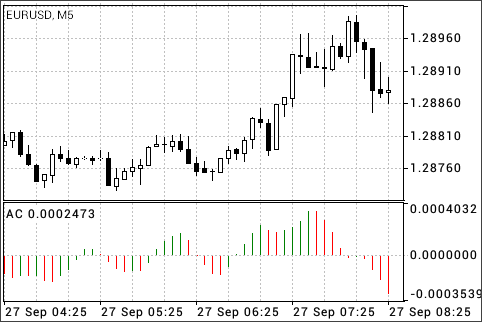Accelerator Oscillator

# Accelerator Oscillator

Price is the latest element to change. Prior to price changes, the market driving force changes its direction, the driving force acceleration must slow down and reach zero. After that it starts accelerating in the opposite direction until price starts changing its direction.

Acceleration/Deceleration Technical Indicator (AC) measures acceleration and deceleration of the current driving force. This indicator will change direction before any changes in the driving force, which, it its turn, will change its direction before the price. If you realize that Acceleration/Deceleration is a signal of an earlier warning, it gives you evident advantages.

The zero line is basically the spot where the driving force is at balance with the acceleration. If Acceleration/Deceleration is above zero, it is usually easier for the acceleration to continue the upward movement (and vice versa in cases when it is below zero). Unlike Awesome Oscillator, zero line cross is not a signal. The only thing that needs to be done to control the market and make decisions is to watch for changes in color. To save yourself serious reflections, you must remember: you can not buy with the help of Acceleration/Deceleration, when the current column is colored red, and you can not sell, when the current column is colored green.

If you enter the market in the direction of the driving force (the indicator is higher than zero, when buying, or it is lower than zero, when selling), then you need only two green columns to buy (two red columns to sell). If the driving force is directed against the position to be opened (indicator below zero for buying or above zero for selling), a confirmation is needed, hence, an additional column is required. In this case the indicator is to show three red columns over the zero line for a short position and three green columns below the zero line for a long position.## Calculation

AC bar chart is the difference between the value of 5/34 of the driving force bar chart and 5-period simple moving average, taken from that bar chart.

MEDIAN PRICE = (HIGH + LOW) / 2

AO = SMA (MEDIAN PRICE, 5) - SMA (MEDIAN PRICE, 34)

AC = AO - SMA (AO, 5)

Where:

MEDIAN PRICE – the median price;
HIGH – the high price of the bar;
LOW – the low price of the bar;
SMA – simple moving average;
AO – Awesome Oscillator.